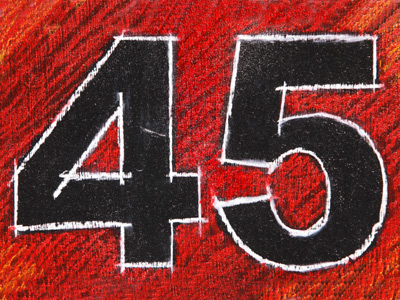5 × 9 = 45.

# 5 Times Table

The five times table is one of the easier times tables to learn - and learn it you must for your 11-Plus exam. One trick that might help you is to multiply the numbers by 10 and then halve them. For example 7 x 5. If you multiply 7 x 10, you get 70. Half of 70 is 35. Therefore 7 x 5 must equal 35 - simple, isn't it?

Five is quite a common number. The Olympic symbol has five rings. Basketball is played with teams of five players. We haves five senses: smell, touch, sight, hearing and taste. There are five elements in Chinese philosophy: water, wood, fire, metal and earth. There are five vowels in the English alphabet: a, e, i, o and u. And the lowest value note is a £5 note.

This may be one of the easiest times tables but don't rush through the questions. A moment of carelessness may lead you to click on the wrong answer by mistake. As with all tests, read the questions properly and choose your answers carefully.

1.
5 × 5 = ?
15
35
45
25
5 is the square root of 25
2.
5 × 11 = ?
65
45
55
35
To multiply 5 x 11, first times 5 by 10 and then add 5
3.
5 × 7 = ?
35
45
25
65
Remember, to multiply any number by 5, first times it by 10 and then halve your answer
4.
5 × 9 = ?
45
54
35
30
To solve this question you could first multiply 5 x 10 and then subtract 5
5.
5 × 4 = ?
20
10
30
40
Another name for the number 20 is a 'score'
6.
5 × 2 = ?
15
12
5
10
To multiply a number by 2 just double it
7.
5 × 6 = ?
25
35
30
24
4 months - April, June, September and November - have 30 days
8.
5 × 3 = ?
20
15
10
25
A rugby union team has 15 players
9.
5 × 8 = ?
30
40
60
20
There are 40 spaces on a Monopoly game board
10.
5 × 12 = ?
50
51
52
60
All numbers in the 5 times table end with either 5 or 0
Author:  Frank Evans